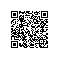# python设计模式（一）：工厂方法与抽象工厂及应用场景

# -*- coding:utf-8 -*-class A:
def __init__(self):
self.word = "运行A"
def run(self):
print(self.word)class B:
def __init__(self):
self.word = "运行B"
def run(self):
print(self.word)def Interface(classname):
"""
工厂模式接口函数
:param classname:
:return:
"""
run = dict(A=A, B=B)
return run[classname]()if __name__ == '__main__':
test1 = Interface('A')
test1.run()
test2 = Interface('B')
test2.run()

结果：
运行A
运行B


# -*- coding:utf-8 -*-class A:
def __init__(self):
self.word = "运行A"
def run(self):
print(self.word)class B:
def __init__(self):
self.word = "运行B"
def run(self):
print(self.word)class Interface:
"""
抽象工厂模式接口类
:param classname:
:return:
"""
def __init__(self, classname=None):
self.test = classname
def run(self):
self.test().run()if __name__ == '__main__':
test1 = Interface()
test1.test = A
test1.run()
test1.test = B
test1.run()
结果：
运行A
运行B使用钉钉扫一扫加入圈子
+ 订阅